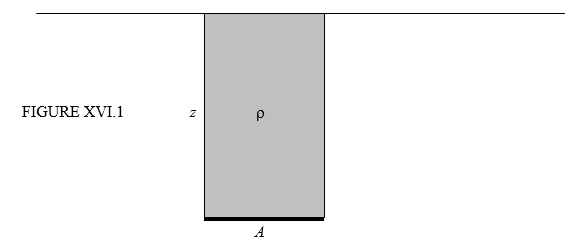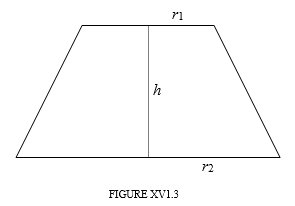$$\require{cancel}$$

16.4: Pressure on a Horizontal Surface. Pressure at Depth

•• Contributed by Jeremy Tatum
• Emeritus Professor (Physics & Astronomy) at University of Victoria

Figure XVI.1 shows a horizontal surface of area $$A$$ immersed at a depth $$z$$ under the surface of a fluid at depth $$z$$. The force $$F$$ on the area $$A$$ is equal to the weight of the superincumbent fluid.This gives us occasion to use a gloriously pompous word. “Incumbent” means “lying down”, so that “superincumbent” is lying down above the area. It is incumbent upon all of us to understand this. The weight of the superincumbent fluid is evidently its volume $$Az$$ times its density $$\rho$$ times the gravitational acceleration $$g$$. Thus

$F=\rho gzA, \label{16.4.1}$

and, since pressure is force per unit area, we find that the pressure at a depth $$z$$ is

$P=\rho gz. \label{16.4.2}$

This is, of course, in addition to the atmospheric pressure that may exist above the surface of the liquid.

The pressure is the same at all points at the same horizontal level within a homogeneous incompressible fluid. This seemingly trivial statement may sometimes be worth remembering under the stress of examination conditions. Thus, let’s look at an example.

Example $$\PageIndex{1}$$

In Figure XVI.2, the vessel at the left is partly filled with a liquid of density 0.8 g cm-3, the upper part of the vessel being filled with air. The liquid also fills the tube along to the point C. From C to B, the tube is filled with mercury of density 13.6 g cm-3. Above that, from B to A, is water of density 1.0 g cm-3, and above that is the atmospheric pressure of 101 kPa.The height of the four interfaces above the thick black line are

• A: 200 cm
• B: 160 cm
• C: 140 cm
• D: 155 cm

With $$g$$ = 9.8 m s-2, what is the pressure of the air in the closed vessel?

Solution

I’ll do the calculation in SI units.

• Pressure at A = 101000 Pa
• Pressure at B = 101000 + 1000 % 9.8 % 0.4 = 104920 Pa
• Pressure at C = 104920 + 13600 % 9.8 % 0.20 = 131576 Pa
• Pressure at D = 131576 - 800 % 9.8 % 0.15 = 130400 Pa,

and this is the pressure of the air in the vessel.

Rather boring so far, and the next problem will also be boring, but the problem after that should keep you occupied arguing about it over lunch.

Exercise $$\PageIndex{1}$$

This problem is purely geometrical and nothing to do with hydrostatics – but the result will help you with the next problem after this. If you don’t want to do it, just use the result in the next problem.Show that the volume of the frustrum of a cone, whose upper and lower circular faces are of radii $$r_{1}$$ and $$r_{2}$$, and whose height is $$h$$, is $$\frac{1}{3}\pi h(r_{1}^{2}+r_{1}r_{2}+r_{2}^{2})$$

Exercise $$\PageIndex{2}$$: Pascal’s ParadoxFigure XVI.4 shows four vessels. The base of each is circular with the same radius, $$\frac{10}{\sqrt{\pi}}$$ cm, so the area is 100 cm2. Each is filled with water (density = 1 g cm-3) to a depth of 15 cm.

Calculate

1. The mass of water in each.
2. The pressure at the bottom of each vessel.
3. The force on the bottom of each vessel.

If the bottom of each vessel were made of glass, which cracked under a certain pressure, which would crack first if the vessels were slowly filled up? If the bottom of each vessel were welded to the scale of a weighing machine, what weight would be recorded?

I’ll leave you to argue about this for as long as you wish.